# How to Choose an Activation Function For Deep LearningThe most vital part of a neural network is its activation functions. Based on them, a neural network decides if a node will be activated or not, which, in turn, determines the efficiency of the network itself. There are different activation functions and which to choose is a common dilemma that data scientists face.

This article will shed light on the different activation functions, their advantages and drawbacks, and which to opt for. But before that, a quick overview of how neural networks operate.

## What is a neural network?

From identifying blurred captchas to reading old manuscripts, our brains can solve the most complex patterns and problems. This ability inspired computer scientists to design artificial neural networks. Like our brains, neural networks compromise neurons or nodes. Each node takes an input, runs operations on it, and produces an output. As we know, the human brain is capable of doing this effortlessly. Neural networks, on the other hand, need to be trained.

Typically, there are three layers involved in training a neural network:

- Input layer: Data is fed to the neural network in this layer. The input could be any raw data, from images to audio. It is passed to nodes without any modification.
- Hidden layer: All data processing is done in this layer. There can be n number of hidden layers in a neural network, depending on how you want to train the system. After computations are performed on data, it is sent to the output layer.
- Output layer: This layer produces the final output.

The diagram below represents a neural network: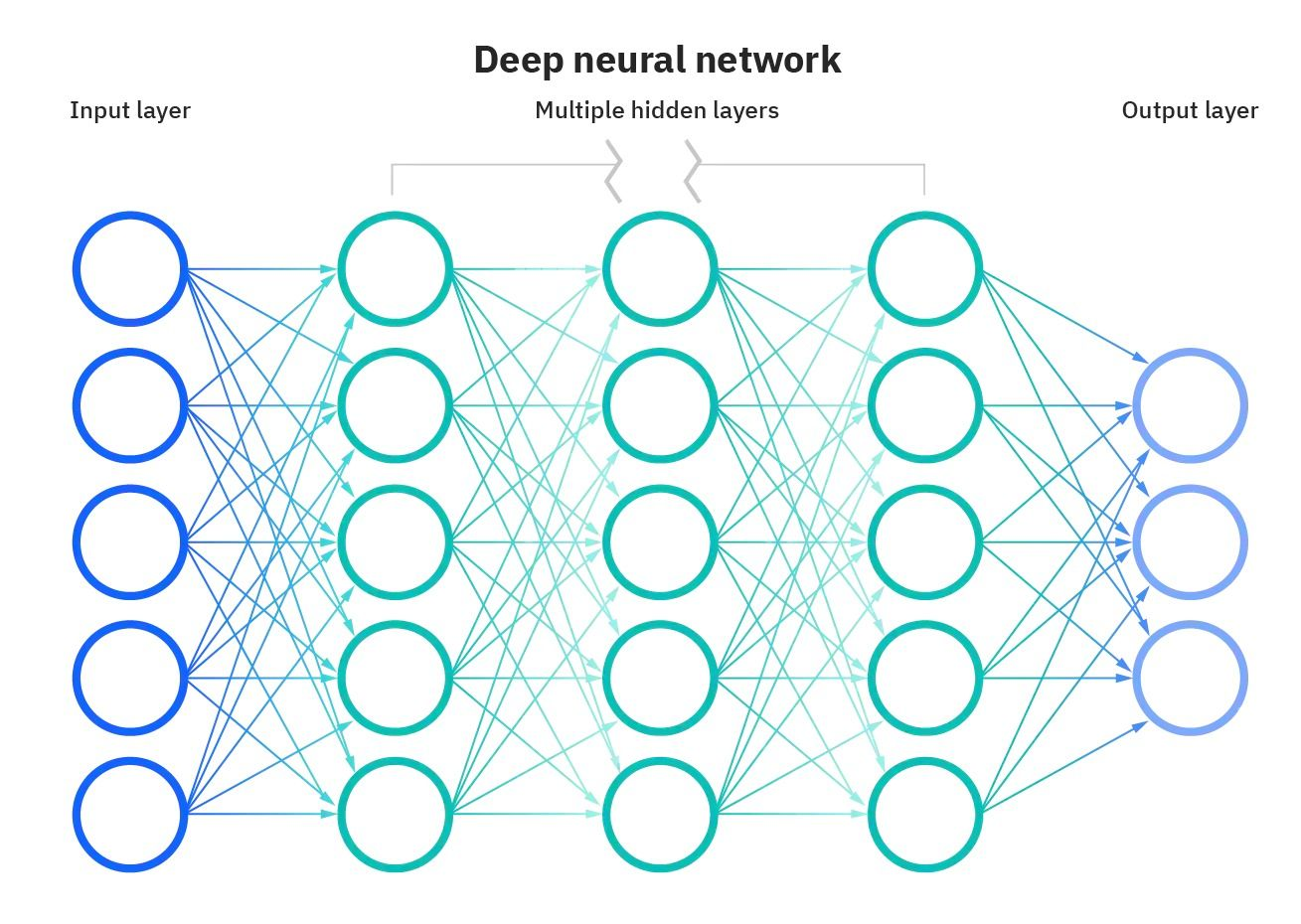Image source: https://www.ibm.com/cloud/learn/neural-networks

Here, each circle represents a node that holds a value ranging from 0 to 1. This number is called 'activation’ and the neuron with the high number activates. Each node connects with all the nodes in the subsequent layer. Depending on the requirement of a neural network, the hidden layer can have n number of nodes and layers. Data transfers from layer to layer and node to node until it reaches the output layer. Nodes with a higher value give the ideal output.

Here’s an example to understand this better:

If your input is a blurred number, the system will divide it into pixels and pass each pixel or activation to one node. Since each number has its shape, the system will first have to identify its edges. After combining them through trial and error, it will match the input to the original number. In this process, only some nodes will get activated.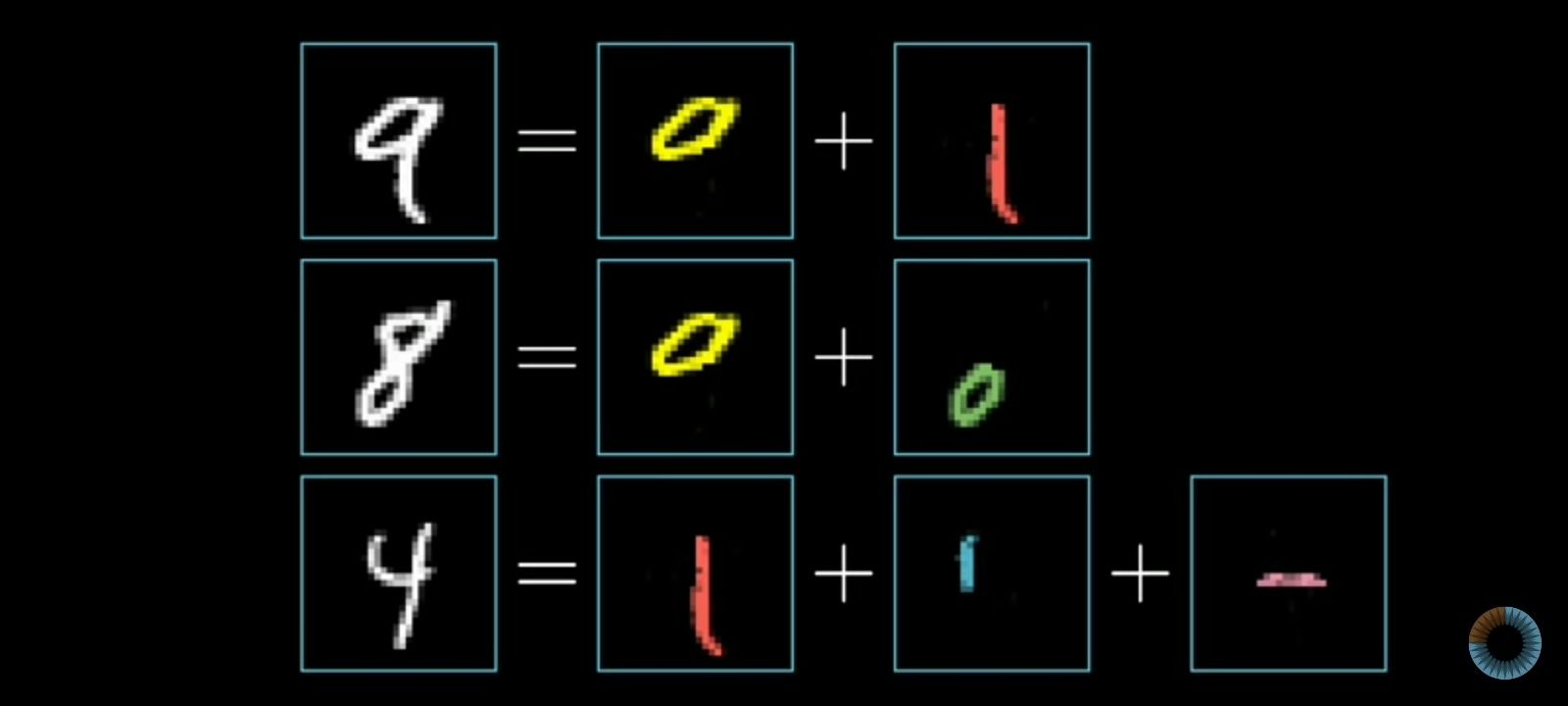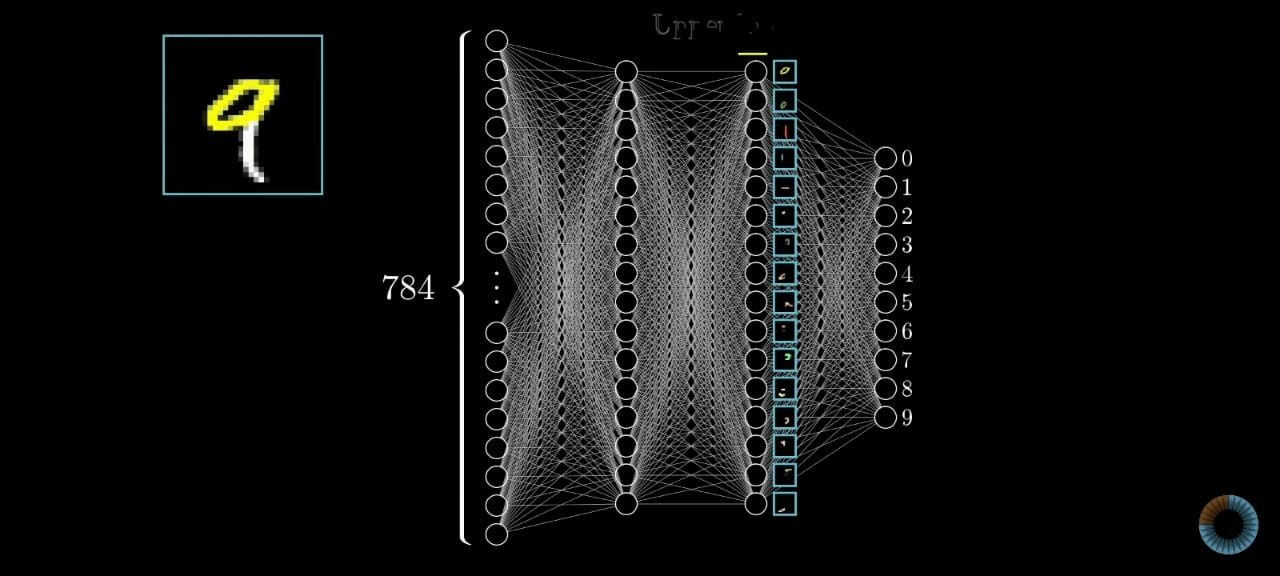Image source: https://www.ibm.com/cloud/learn/neural-networks

The question remains: which nodes get activated and which don’t? And what decides this? Here is where the role of activation functions becomes significant.

## What is an activation function?

An activation function is a mathematical equation that determines whether a node should be activated or not. If a node is activated, it will pass data to the nodes of the next layer. The activation function can be calculated by multiplying input and weight and adding a bias.

Mathematically, it can be represented as:

Z = Activation function(∑ (weights*input + bias))

So, if inputs are x1+x2+x3….xn and the weights are w1+w2 + w3.......wn

then, the activation would be (Activation function (x1w1+x2w2+x3w3……xnwn) +bias)

Weights are the coefficients of the equation being worked on. Bias is a constant value added to the product of input and weights, so that the value of the output can be more towards the positive or negative side.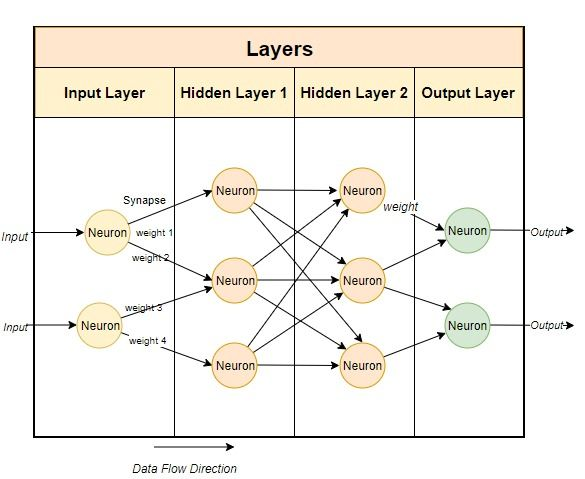Image source: https://medium.com/fintechexplained/neural-networks-bias-and-weights-10b53e6285da

The purpose of the activation function is to add nonlinearity to a neural network. There’s a lot of trial and error when training a neural network to obtain an output. Therefore, it’s necessary to update the weights and biases of neural networks, known as backpropagation. This is possible due to activation functions as they send gradients to correct the weights and biases of neurons.

## Types of activation functions

### Binary step function

Mathematical expression: f(z) = 0 for z < 0, 1 for z ≥ 1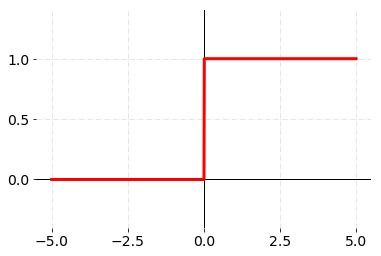Source: Medium

The step function is also known as the threshold function. This is because a node’s capacity to be activated or not depends on the function’s capacity to surpass a threshold value. If its value is above the threshold value, it will activated. If not, it won’t. It’s ideal for solving complex patterns because it can’t provide multi-value outputs. Besides, the gradient is always zero, which doesn’t help in backpropagation.

### Sigmoid

Mathematical expression: f(z) = 1/(1+e⁻z)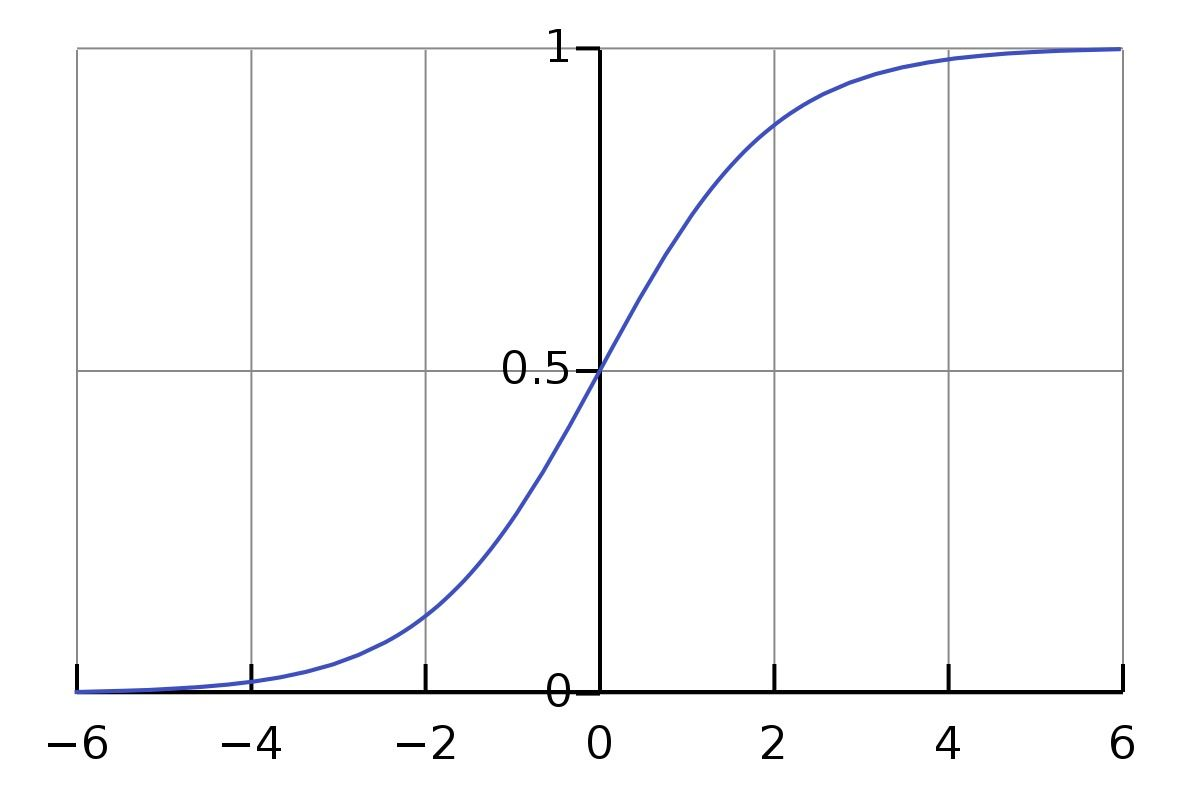Image source: https://en.wikipedia.org/wiki/Sigmoid_function

The sigmoid function is a nonlinear function used in regression models. Its graph resembles an ‘S’. This function converts its input into a probability value between 0 and 1. Large negative values are converted towards 0 while large positive values are converted towards 1.

This function, which is computationally expensive, isn’t used in the hidden layers of a convolutional neural network. Since it gives a low gradient for values greater than 3 and less than -3, the network fails to learn and perform. This is called the vanishing gradient problem.

### Tanh

Mathematical expression: f(x) = a =tanh(z) =(ez - e⁻z)/(ez +e⁻z)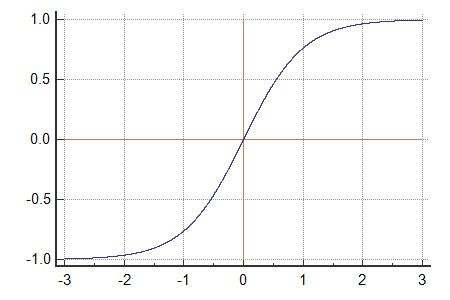Image source: https://www.medcalc.org/manual/tanh-function.php

The tanh function is best suited for the classification of two different classes. Like sigmoid, it’s nonlinear and also forms an S-shaped graph. Its range falls between -1 and 1. Being zero-centric in nature, it has a high optimization speed. For large positive inputs, it gives an output closer to 1 and for larger, negative inputs, the output is closer to -1. The function isn’t used in the output layer.

### ReLu

Mathematical expression: f(z) = max(0,z)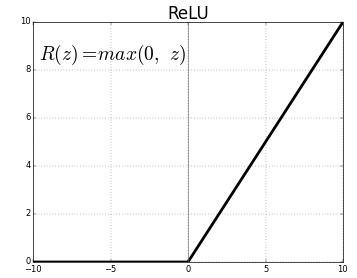ReLu, an alternative to both sigmoid and tanh activation functions, is one of the most widely activated in convolutional neural networks and deep learning. It doesn’t have a vanishing gradient problem unlike sigmoid and tanh. The range falls between 0 and + infinity.

The ReLu function performs faster calculations because it does not use exponential terms. The drawback, however, is that its positive side can go to the higher end which leads to computation problems during the training phase.

### Softmax

Mathematical expression: f(x) = eˣᵢ / (Σⱼ₌₀ eˣᵢ)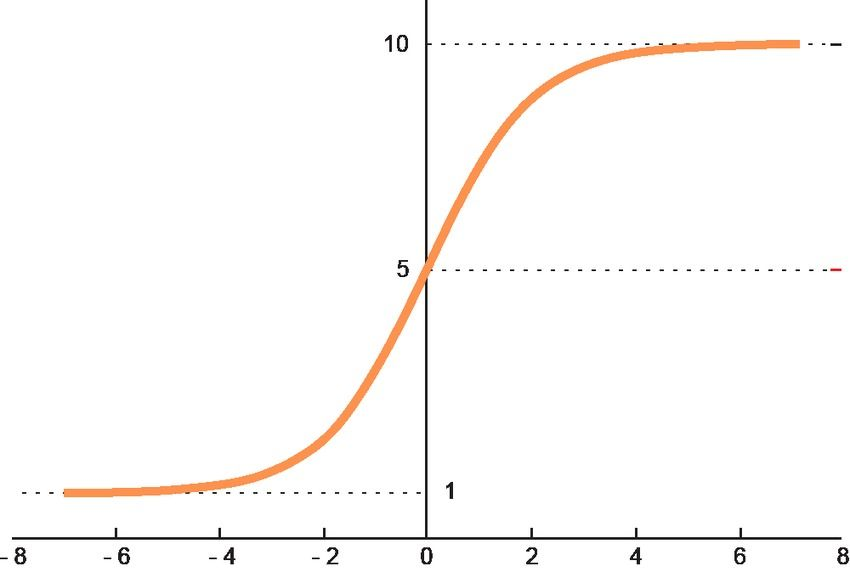Image source: https://www.researchgate.net/figure/Graphic-representation-of-the-softmax-activation-function_fig5_348703101

Softmax is a nonlinear function used to handle multiple classes. It adjusts the outputs for each class between 0 and 1, then divides them by their sum to determine the likelihood of the input value falling into a specific category of classes. The function is predominantly used in the output layer, specifically for neural networks that require the classification of inputs in multiple categories.

## Choosing the right activation function for a neural network

There’s no clear-cut answer to selecting an activation function as it all depends on the problem to be solved. But if you’re new to deep learning, you might want to start with the sigmoid function before moving on to others - depending on the outcome of your results.

Generally, problems are of two types: regression and classification. The linear activation function is convenient for regression problems, while nonlinear functions are more suitable for classification problems.

Tip: Use the sigmoid function for binary classification and softmax activation for multiclass classification.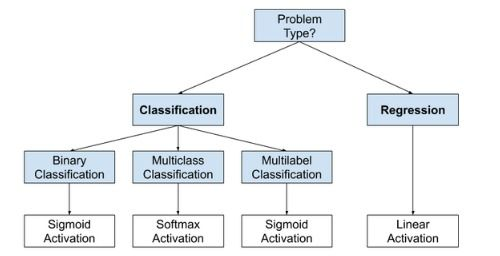Image source: https://www.kaggle.com/general/212325

• For hidden layers, a differential nonlinear function is more suitable because it trains the neural network model to work on more complex problems.
• Always use the ReLu function in hidden layers.
• The softmax function is typically used for the output layer.
• If you’re confused about which activation function to use, begin with the ReLu function as it’s used in most scenarios these days.### Press

What's up with Turing? Get the latest news about us here.### Blog